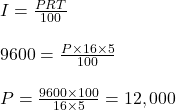## Frans paid R9600 as interest on a loan he took 5 years ago at 16% rate. What’s was the amount he took as loan?

Question

Frans paid R9600 as interest on a loan he took 5 years ago at 16% rate. What’s was the amount he took as loan?

in progress 0
2 months 2021-08-04T09:12:42+00:00 2 Answers 2 views 0

The amount he took as loan = R12,000

Step-by-step explanation:

Simple Interest

Let loan amount be = P

R = 16%

T = 5years

I = 9600

Find P2. Step-by-step explanation:

Interest (I)=R9600

Rate(R)=16%

Time (T)=5years

Principal (P)=?

P=100×I÷R×T

P=100×R9600÷16×5

P=R960000÷80

P=R12, 000